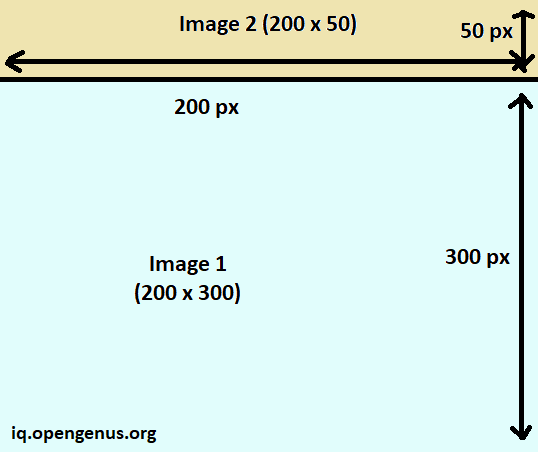# Combine two images horizontally and vertically in PythonIn this article, we have explained how to Combine two images horizontally and vertically in Python using Numpy / OpenCV.

1. Combine two images vertically
2. Combine two images horizontally

These technique will be using Numpy library but will work with OpenCV functions as well. Combine images and then, apply OpenCV.

## Combine two images vertically

To combine two images vertically, the width of both images should be same. If the width is different, then one of the images must be resized to make the width same.

Once the width of both images are same, then we can use the np.concatenate function of Numpy with axis as 0.

The first parameter will be the top image and the second parameter will be the bottom image. Both images should be loaded as Numpy array which can be done using np.load().

This is the main code statement:

``````final_image = np.concatenate((image_top, image_original), axis=0)
``````

Following is the complete Python code to combine two images vertically:

``````import numpy as np

# let shape be (200 x 300 x 3) HWC

height_part1 = 50
width_part1 = 200 # should be same as width of image_original
channels = 3 # for RGB images

image_top = np.zeros((height_part1, width_part1, channels), np.uint8)
final_image = np.concatenate((image_top, image_original), axis=0)
``````

Following is the visual image combining 2 images vertically:## Combine two images horizontally

To combine two images horizontally, the height of both images should be same. If the height is different, then one of the images must be resized to make the height same.

Once the height of both images are same, then we can use the np.concatenate function of Numpy with axis as 1. Note, for combining vertically, the axis was 0.

The first parameter will be the left image and the second parameter will be the right image. Both images should be loaded as Numpy array which can be done using np.load().

This is the main code statement:

``````final_image = np.concatenate((image_top, image_original), axis=1)
``````

Following is the complete Python code to combine two images horizontally:

``````import numpy as np